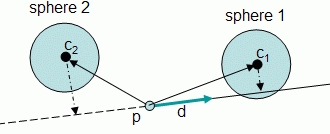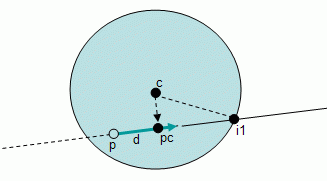Prev: Plane Next: Ray-Triangle Intersection

Given a ray, defined by a point and a unit vector, and a sphere do they intersect? If so where? Do they intersect in a single point, or in two points? In the figure below we can see several possibilities.The first question is whether the ray intersects the sphere or not. In order to find out, the distance between the center of the sphere and the ray must be computed. If that distance is larger than the radius of the sphere then there is no intersection.

To compute the distance two cases are considered: either the center of the sphere projects on the ray, or it doesn’t. A simple dot product will do the trick.

Let v be the vector that goes from p to c (the center of the sphere). Then if

• d . v > 0 // the distance to the ray can be computed (Sphere 1)
• d . v <= 0 // the sphere is behind the ray (Sphere 2)
•Case 1: The center of the sphere projects on the ray

The following figure shows the three possible cases: no intersection (sphere A), single intersection (sphere B), and two intersections (sphere C).First compute the projection of the center of the sphere on the ray. Let pc be that projection.

If the distance from pc to the ray is greater than the ray then there is no intersection (sphere A in the above figure).

If the distance from pc to the ray is equal to the radius of the sphere, then the intersection is a single point: pc (sphere B).

The former case requires a little bit extra work.The point pc has already been found. So the task at hand is to find i1 and/or i2. The following procedure gives us i1, and finding i2 is left as an (easy) exercise to the reader.

If the distance from p to i1 is known, lets called di1, then finding i1 is achieved with the following equation:

`    `	i1 = p + d * di1``

Hence all there is left to do is to find di1.

The triangle defined by i1, pc and c is a right triangle (i.e. on of the angles is 90 degrees), hence the Pythagorean theorem applies. Let

```        a = |c - i1| = radius
b = |pc - c|
c = |pc - i1|```

The only unknown is c, and it can be computed as:

`	c^2 = a^2 - b^2`

Then

`	di1 = | pc - p | - c`

The previous computation of di1 assumed that the origin of the ray was not inside the sphere. If p is inside the sphere then the computation of di1 is slightly different:

`	di1 = | pc - p | + c`Case 2: The center of the sphere is behind the ray

If it is assumed that the origin of the ray is outside the sphere then there is no possible intersection. Otherwise, there is an intersection if the distance from p to c is less than or equal to the radius. If is equal then the intersection is the point p itself.

If the origin of the ray is inside the sphere a similar reasoning to the case presented previously may be applied. Note that the projection is done on the line, and not on the ray. The following figure shows the relevant points involved.Computing the distance from pc to i1 is exactly the same as before. Let dist be that distance. The value of di1 can be computed as:

`	di1 = dist - | pc - p |`

Algorithm

So here it is the full algorithm to compute the intersection between a ray and a sphere. It is assumed that the ray is defined by an origin p, and a direction d (unit vector), and that the sphere has a center c, and a radius r.

```
vpc = c - p  // this is the vector from p to c

if ((vpc . d) < 0) // when the sphere is behind the origin p
// note that this case may be dismissed if it is
// considered that p is outside the sphere
if (|vpc| > r)

// there is no intersection

else if (|vpc| == r)

intersection = p

else // occurs when p is inside the sphere

pc = projection of c on the line
// distance from pc to i1
dist = sqrt(radius^2 - |pc - c|^2)
di1 = dist - |pc - p|
intersection = p + d * di1

else // center of sphere projects on the ray

pc = projection of c on the line
if (| c - pc | > r)

// there is no intersection

else
// distance from pc to i1
dist = sqrt(radius^2 - |pc - c|^2)

if (|vpc| > r) // origin is outside sphere

di1 = |pc - p| - dist

else // origin is inside sphere

di1 = |pc - p| + dist

intersection = p + d * di1```

 Prev: Plane Next: Ray-Triangle Intersection

### 5 Responses to “Ray-Sphere Intersection”

1.Thanks for the tutorial, very simple and easy to understand.

Small correction, when you say:
“If the distance from pc to the ray is greater than the ray then there is no intersection (sphere A in the above figure).”

Shouldn’t it be:
“If the distance from pc to the ray is greater than the radius of the sphere then there is no intersection (sphere A in the above figure).”

?

2.For the case where you have two intersections of the sphere
to the line: If the angle at c-pc to the line denoted by
p->d is always 90 degrees, then isn’t the distance from i1
to pc always identical to the distance from pc to i2?

3.Not sure what you mean by this: “If the distance from pc to the ray is greater than the ray then there is no intersection.”

Did you perhaps mean “If the distance from pc to the center of the sphere is greater than the radius then there is no intersection.”?

4.Everything is good, and the tutorial is perfect except for the projected point on the line

pc you never say how to calculate it and without it the algoritm cant be used

•Hi Jakob,

Thanks for the feedback. pc is the projection of the center of the sphere on the ray segment. There is a link in the text to the page that describes the projection operation, that’s why I didn’t mention it in here.

This site uses Akismet to reduce spam. Learn how your comment data is processed.